Author

Emmanouil Tranos

Published

November 11, 2022

## Street netorks

Until this point the focus has been on networks between cities. Now we are turning the focus on networks within cities and more specifically on street networks. These are spatial networks whose edges and not only their nodes contain spatial information. To summarise, in spatial or, in other words, geospatial networks:

• Nodes always contain geographical coordinates.

• However, edges can:

• either contain geographical information (e.g. road networks),

• or not, and instead just link two nodes (e.g. social networks whose node’s geograpical location is known to us)

Street network analysis is not new. For instance, Leonhard Euler proved that the Seven Bridges of Königsberg problem had no solution. This type of problems and analysis – routing – later on became integral part of the standard GIS toolkit.

Why is it important to study street networks:

• To understand the form of cities. There are quite a few recent studies (e.g. Boeing 2019, 2017), but this was also part of an older strand of research called Space Syntax .

• To understand the structure of transportation systems and support transportation planning.

Some key characteristics of street networks:

• They might have multiple edges between two nodes.

• Street networks are approximately planar .

• Edges contain geographical information.

Topological measures are important to understand and (maybe compare different) urban street networks.

Try to think what the below topological measures might mean for a street network:

• Node density

• Node eccentricity

• Network diameter

• Node degree

• Clustering coefficient

• Betweenness centrality and its maximum value in a network

• Closeness centrality

Key development: OpenStreetMap. Known as the Wikipedia for maps, OSM is a collaborative, citizen science project, which allows individuals to contribute in building openly distributed geographic data . Through OSM urban analysts have access to street network data from around the world, something which wasn’t the case, let’s say, 20 years ago.

The below are based on the very helpful vignettes of the `sfnetworks` and `osmdata` packages. Please make sure to revisit these materials on your own time.

``````library(sfnetworks) #tidy geospatial networks
library(sf)         #simple features, spatial data
library(tidygraph)  #tidyverse's version of igraph
library(tidyverse)  #you should know this by now
library(igraph)     #as above
library(osmdata)    #access OSM data
library(dbscan)     #this is the dbscan clustering algorithm

knitr::opts_chunk\$set(echo = TRUE) ``````

In geospatial networks:

• nodes always contain geographical coordinates (`sf` objects),

• edges can:

• either contain geographical information (e.g. road networks)

• or not, and instead just link two nodes (e.g. geospatial social networks)

## A first ‘toy’ example

The below code chunks create and manipulate a toy geospatial network.

``````p1 = st_point(c(7, 51))
p2 = st_point(c(7, 52))
p3 = st_point(c(8, 52))
p4 = st_point(c(8, 51.5))

l1 = st_sfc(st_linestring(c(p1, p2)))
l2 = st_sfc(st_linestring(c(p1, p4, p3)))
l3 = st_sfc(st_linestring(c(p3, p2)))

edges = st_as_sf(c(l1, l2, l3), crs = 4326)
nodes = st_as_sf(c(st_sfc(p1), st_sfc(p2), st_sfc(p3)), crs = 4326)

edges\$from = c(1, 1, 3)
edges\$to = c(2, 3, 2)``````

As you can see below, `net` has a valid spatial network structure

``net = sfnetwork(nodes, edges)``
``Checking if spatial network structure is valid...``
``Spatial network structure is valid``

We can check it’s class:

``class(net)``
`` "sfnetwork" "tbl_graph" "igraph"   ``

And check it characteristics.

``net``
``````# A sfnetwork with 3 nodes and 3 edges
#
# CRS:  EPSG:4326
#
# A directed acyclic simple graph with 1 component with spatially explicit edges
#
# Node Data:     3 x 1 (active)
# Geometry type: POINT
# Dimension:     XY
# Bounding box:  xmin: 7 ymin: 51 xmax: 8 ymax: 52
x
<POINT [°]>
1      (7 51)
2      (7 52)
3      (8 52)
#
# Edge Data:     3 x 3
# Geometry type: LINESTRING
# Dimension:     XY
# Bounding box:  xmin: 7 ymin: 51 xmax: 8 ymax: 52
from    to                    x
<int> <int>     <LINESTRING [°]>
1     1     2         (7 51, 7 52)
2     1     3 (7 51, 8 51.5, 8 52)
3     3     2         (8 52, 7 52)``````

Just like `igraph` we can refer to nodes using the `names` we can assign to them.

``````nodes\$name = c("city", "village", "farm")
edges\$from = c("city", "city", "farm")
edges\$to = c("village", "farm", "village")

edges # check the from and to columns ``````
``````Simple feature collection with 3 features and 2 fields
Geometry type: LINESTRING
Dimension:     XY
Bounding box:  xmin: 7 ymin: 51 xmax: 8 ymax: 52
Geodetic CRS:  WGS 84
x from      to
1        LINESTRING (7 51, 7 52) city village
2 LINESTRING (7 51, 8 51.5, 8... city    farm
3        LINESTRING (8 52, 7 52) farm village``````
``net = sfnetwork(nodes, edges, node_key = "name")``
``Checking if spatial network structure is valid...``
``Spatial network structure is valid``

`net`’s edges contain geographical information. So, let’s create an `other_net` whose edges do not contain geographical information. This could have been a geospatial social network, for example. We know where each node is located in space and then the edges illustrate the existence of a connection between two nodes. To do so, we simply set the `st_geometry` equal to `NULL`. The plots below will make these differences clear.

``````st_geometry(edges) = NULL

other_net = sfnetwork(nodes, edges, edges_as_lines = TRUE)``````
``Checking if spatial network structure is valid...``
``Spatial network structure is valid``

We can actually plot both example.

``plot(net, cex = 2, lwd = 2, main = "Original geometries")````plot(other_net, cex = 2, lwd = 2, main = "Geospatial social network example")``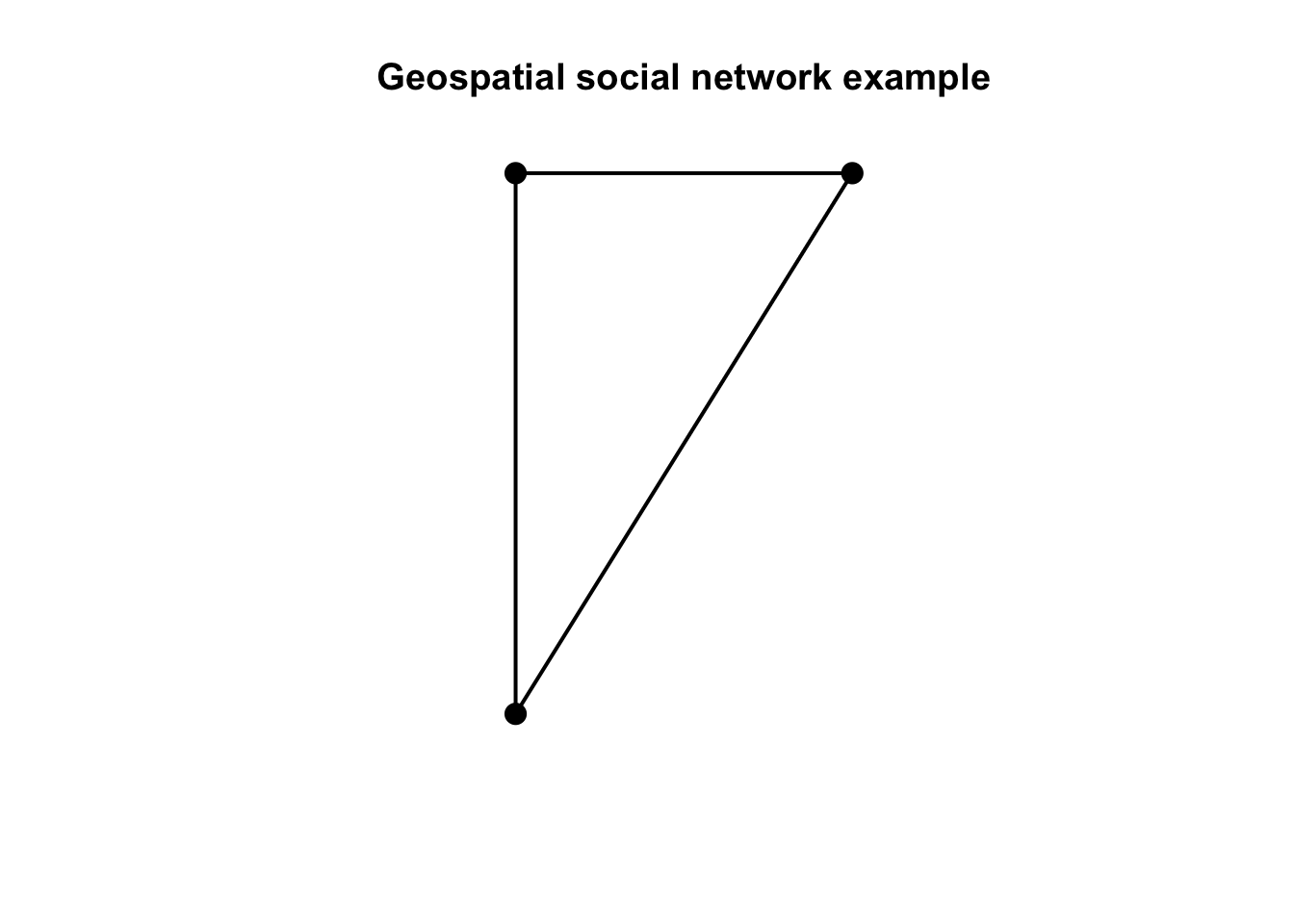## A more ‘real’ street network

Let’s increase the complexity now. We can use the `roxel` data from the `sfnetworks` package. Roxel is a small town just outside Munster in Germany.

The `as_sfnetwork()` function converts the spatial object to a network. So, this is the spatial object and this is how it’s geometry looks like.

``roxel``
``````Simple feature collection with 851 features and 2 fields
Geometry type: LINESTRING
Dimension:     XY
Bounding box:  xmin: 7.522594 ymin: 51.94151 xmax: 7.546705 ymax: 51.9612
Geodetic CRS:  WGS 84
# A tibble: 851 x 3
name                  type                                           geometry
* <chr>                 <fct>                                  <LINESTRING [°]>
1 Havixbecker Strasse   residential      (7.533722 51.95556, 7.533461 51.95576)
2 Pienersallee          secondary   (7.532442 51.95422, 7.53236 51.95377, 7.53~
3 Schulte-Bernd-Strasse residential (7.532709 51.95209, 7.532823 51.95239, 7.5~
4 <NA>                  path        (7.540063 51.94468, 7.539696 51.94479, 7.5~
5 Welsingheide          residential       (7.537673 51.9475, 7.537614 51.94562)
6 <NA>                  footway     (7.543791 51.94733, 7.54369 51.94686, 7.54~
7 <NA>                  footway           (7.54012 51.94478, 7.539931 51.94514)
8 <NA>                  path        (7.53822 51.94546, 7.538131 51.94549, 7.53~
9 <NA>                  track       (7.540063 51.94468, 7.540338 51.94468, 7.5~
10 <NA>                  track       (7.5424 51.94599, 7.54205 51.94629, 7.5419~
# ... with 841 more rows``````
``plot(st_geometry(roxel))``Now, we can convert it to a geospatial network and plot it.

``````net <- as_sfnetwork(roxel)
plot(net)``````Instead of `plot()` we can also use `ggplot2` functions. `autoplot()` does what it says.

``````autoplot(net) +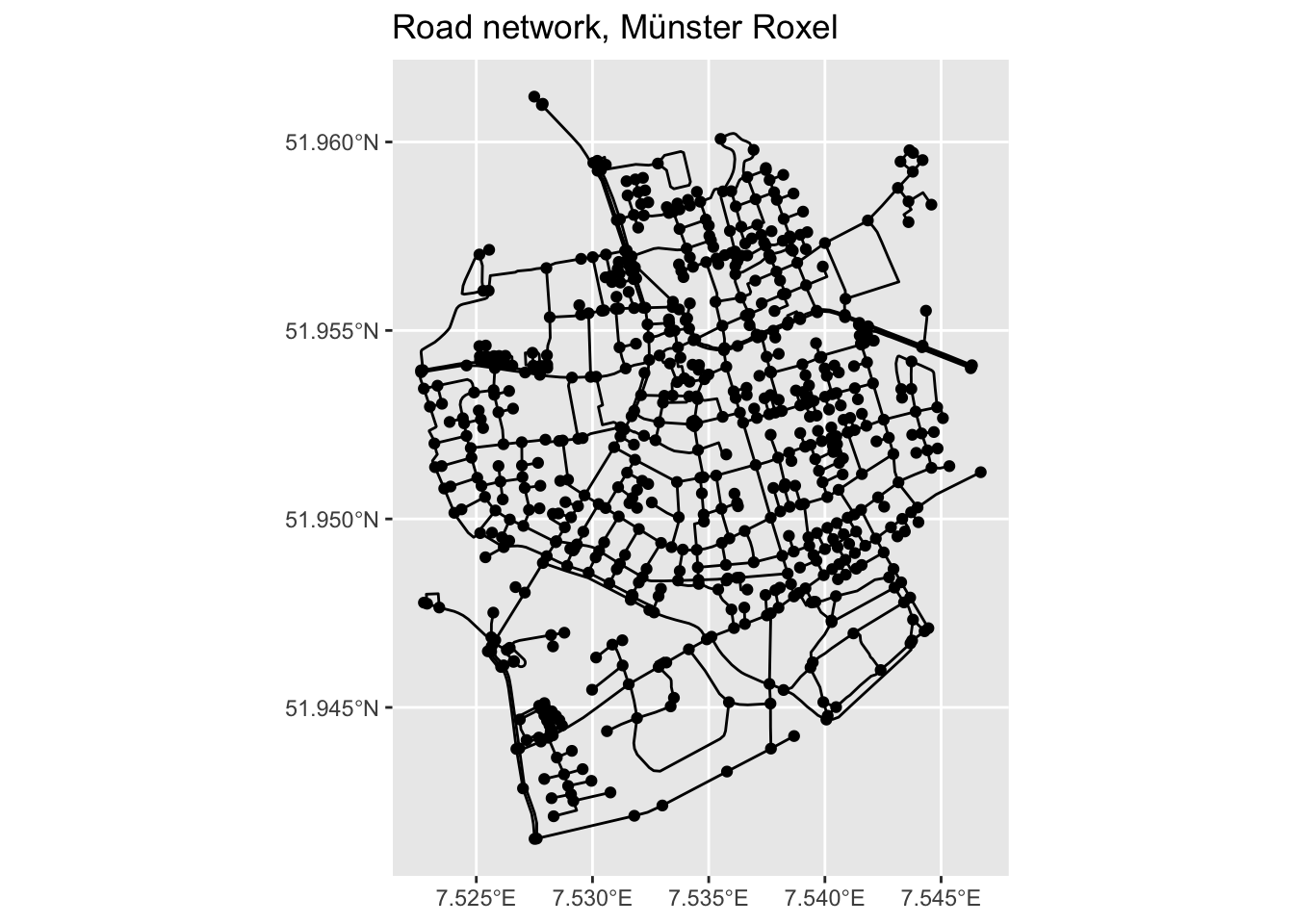We have a network, so let’s do some network analysis.

First thing we need to do is to activate the network component we are interested in. In the below example we want to calculate the betweenness centrality of nodes and, therefore, we need to first activate the nodes.

So, the `net` object now has a `bc` column in the node attributes

``````net = net %>%
activate("nodes") %>%
mutate(bc = centrality_betweenness()) %>%
print() # print() is equal to just run the object, in this case net.``````
``````# A sfnetwork with 701 nodes and 851 edges
#
# CRS:  EPSG:4326
#
# A directed multigraph with 14 components with spatially explicit edges
#
# Node Data:     701 x 2 (active)
# Geometry type: POINT
# Dimension:     XY
# Bounding box:  xmin: 7.522622 ymin: 51.94151 xmax: 7.546705 ymax: 51.9612
geometry     bc
<POINT [°]>  <dbl>
1 (7.533722 51.95556) 12936.
2 (7.533461 51.95576) 11824
3 (7.532442 51.95422) 11926.
4  (7.53209 51.95328)  7259.
5 (7.532709 51.95209)  5668
6 (7.532869 51.95257)  2374
# ... with 695 more rows
#
# Edge Data:     851 x 5
# Geometry type: LINESTRING
# Dimension:     XY
# Bounding box:  xmin: 7.522594 ymin: 51.94151 xmax: 7.546705 ymax: 51.9612
from    to name                  type                                geometry
<int> <int> <chr>                 <fct>                       <LINESTRING [°]>
1     1     2 Havixbecker Strasse   residential (7.533722 51.95556, 7.533461 51~
2     3     4 Pienersallee          secondary   (7.532442 51.95422, 7.53236 51.~
3     5     6 Schulte-Bernd-Strasse residential (7.532709 51.95209, 7.532823 51~
# ... with 848 more rows``````

We can now create a map of this spatial network, the size and the colour of its nodes are based on their betweenness centrality.

``````ggplot() +
geom_sf(data = st_as_sf(net, "edges"), col = "grey50") +
geom_sf(data = st_as_sf(net, "nodes"), aes(col = bc), size = 1.5) +
ggtitle("Betweenness centrality in Münster Roxel")``````To better understand the structure of the above `ggplot()` look up `geom_sf()` and `st_as_sf`. If you only run `net` and `st_as_sf(net, "edges")` you can see what was the type of `net` originally and what it was transformed to with the `st_as_sf`.

Question: Can you interpret betweenness centrality in this context?

If we want to get rid of geometries, all we need to do is to remove the geometry. As per base `R`, setting something equal to `NULL` means that we remove it.

``````net %>%
activate("edges") %>%
st_set_geometry(NULL) %>%
plot(draw_lines = T, main = "Edges without geometries")``````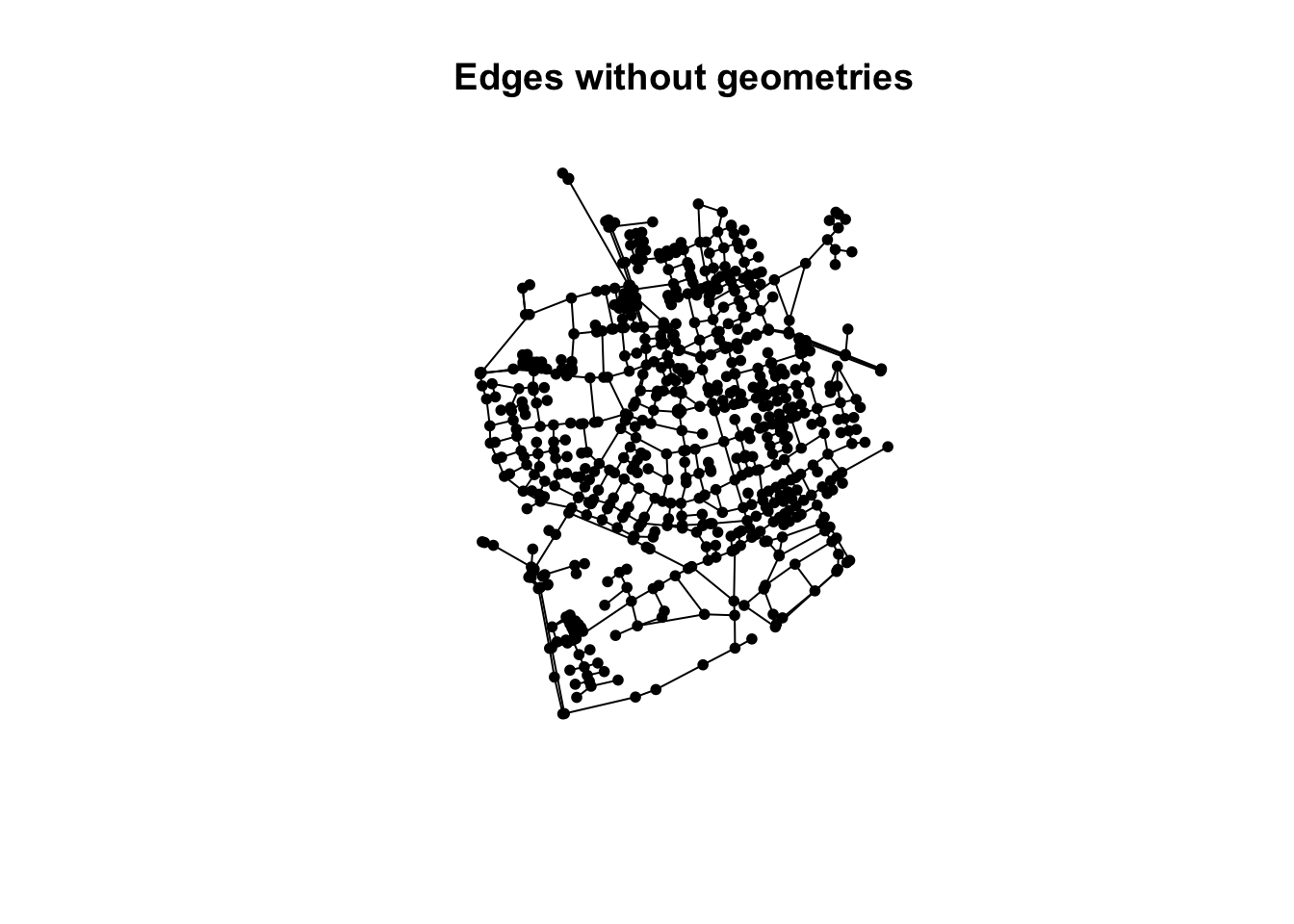``````net %>%
activate("nodes") %>%
st_set_geometry(NULL) %>%
plot(vertex.color = "black", main = "Nodes without geometries")``````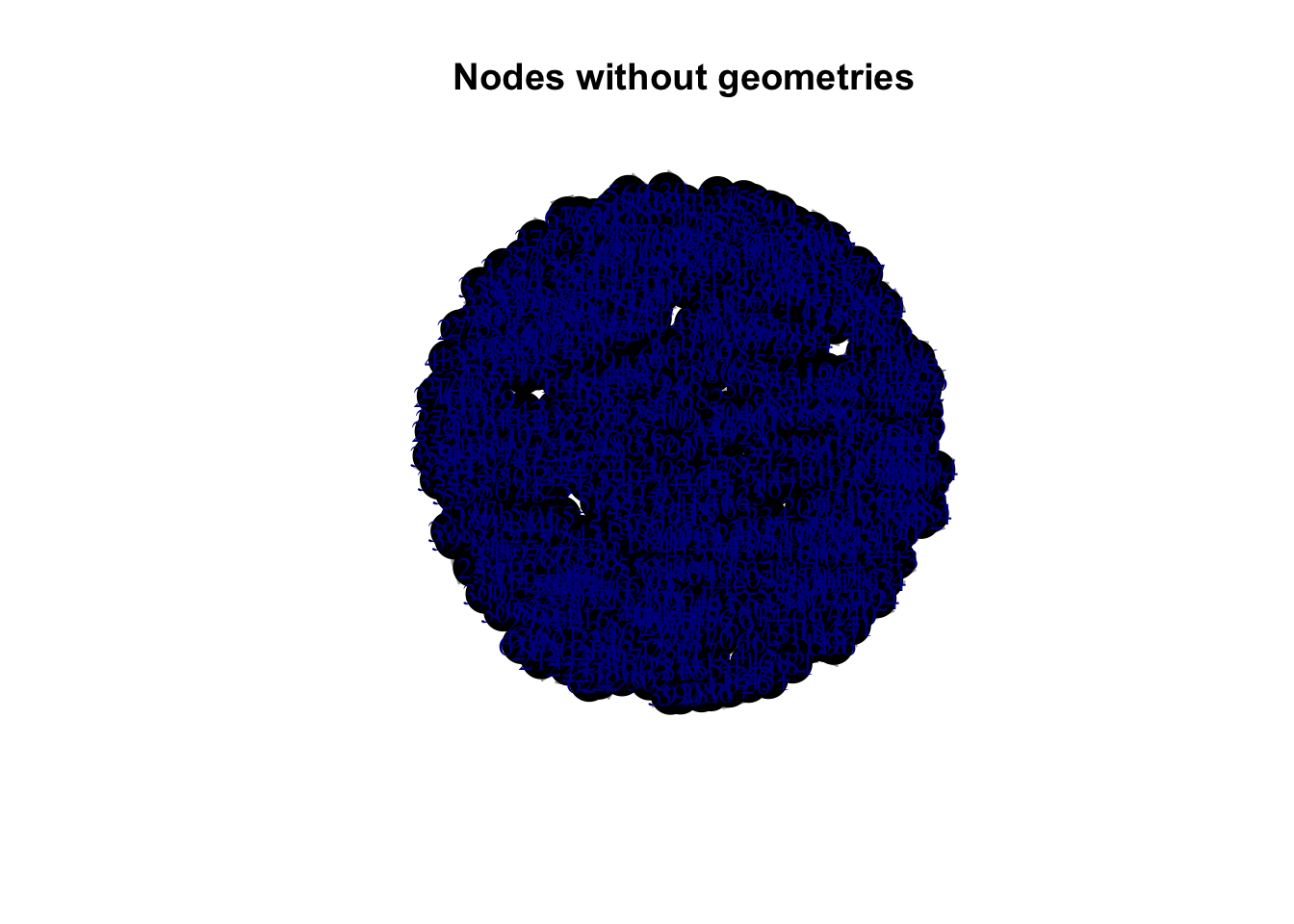## A real world example from Open Street Map

Let’s now try to use some real-world data from OSM. The below code extracts the Bristol street data from the OSM API.

To understand the `key` and `value` options, go to OSM’s wiki page.

Keep an eye on the below. Depending on your internet connections and the OSM’s API response time, it might take a couple of minutes. If you get an error message from running the `opq()` try again, it might work the second time as something might have gone wrong the first one. This is not uncommon when we extract data over the internet through an API. Usually such API requests are wrapped up in a `if_else()` function to re-try if the first attempt was unsuccessful.

``````bristol_data <- opq(bbox = 'Bristol') %>%
key = 'highway',
) %>%
osmdata_sf(quiet = FALSE)``````
``Issuing query to Overpass API ...``
``Announced endpoint: none``
``Query complete!``
``converting OSM data to sf format``
``````# extract the roads

# build the sfnetwork object
bristol_sfn <- as_sfnetwork(bristol_roads, directed = FALSE, length_as_weight = TRUE)

ggplot() +
geom_sf(data = st_as_sf(bristol_sfn, "edges"), col = "grey50") +
geom_sf(data = st_as_sf(bristol_sfn, "nodes"), size = .01)``````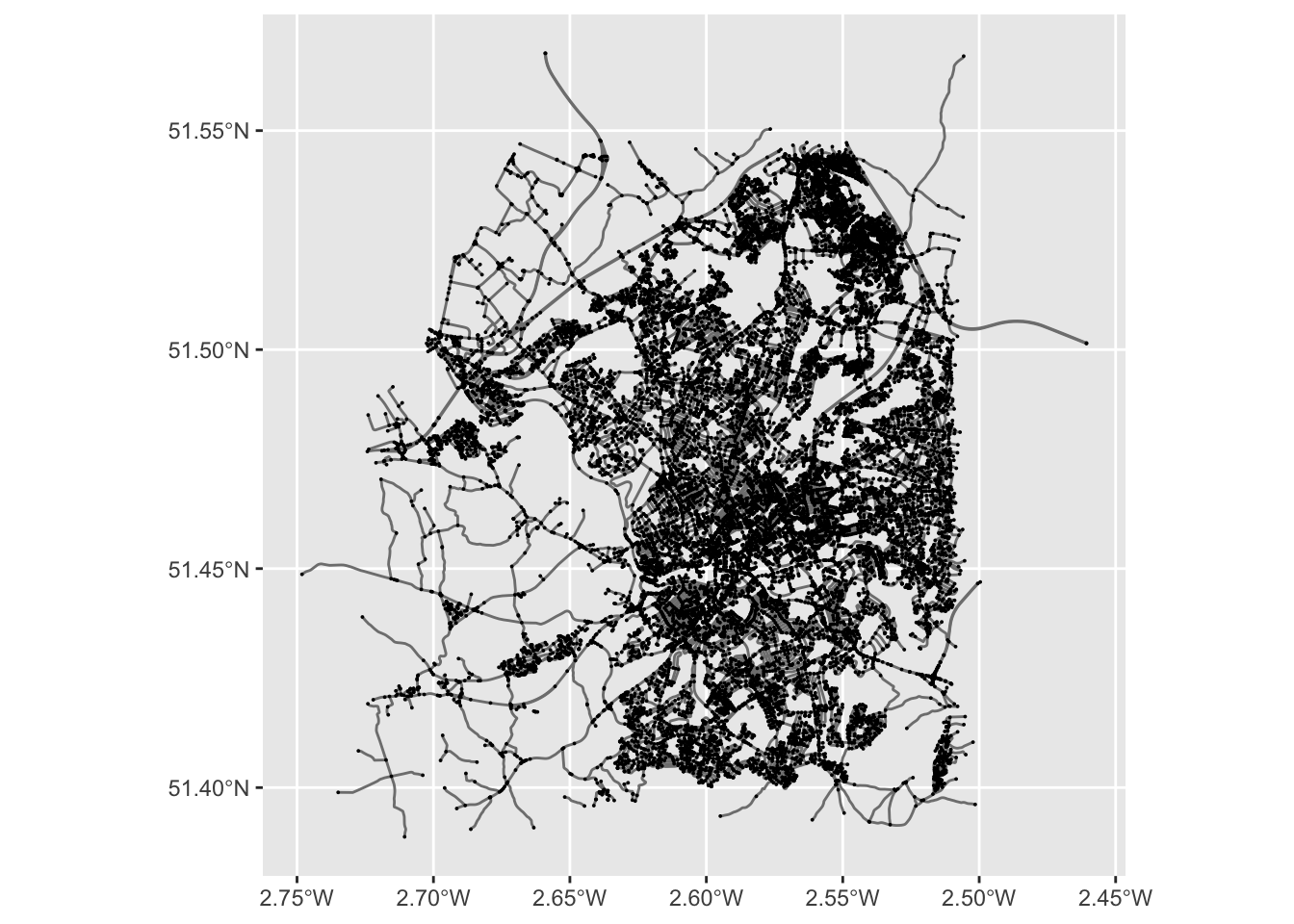Network data wrangling. We need to:

1. Remove multiple edges and loops. AKA simplifying the networks.
1. Remove nodes that are interior points in a line and define the shape of the line. AKA smoothing the network.

To keep track of the changes, let’s keep a note of the original network size.

``````# size of original network
gsize(bristol_sfn)  # number of edges``````
`` 15104``
``gorder(bristol_sfn) # number of nodes``
`` 20915``

Now we can simplify the network.

``````bristol_wrangled <- bristol_sfn %>%
activate("edges") %>%
filter(!edge_is_multiple()) %>%   # Simplify the network
filter(!edge_is_loop())           # Simplify the network

# size of simplified network
gsize(bristol_wrangled)  # number of edges``````
`` 14904``
``gorder(bristol_wrangled) # number of nodes``
`` 20915``

Remove the interior points…

``````bristol_wrangled <- convert(bristol_wrangled, to_spatial_smooth)

# size of simplified network
gsize(bristol_wrangled)  # number of edges``````
`` 11747``
``gorder(bristol_wrangled) # number of nodes``
`` 17758``

… and plot the network again.

``````ggplot() +
geom_sf(data = st_as_sf(bristol_wrangled, "edges"), col = "grey50") +
geom_sf(data = st_as_sf(bristol_wrangled, "nodes"), size = .01)``````## Network measures

Now we can calculate betweenness centrality for Bristol, using the OSM street network we acquired.

``````bristol_wrangled <- bristol_wrangled %>%
activate("nodes") %>%
mutate(bc = centrality_betweenness())``````
``````Warning in betweenness(graph = graph, v = V(graph), directed = directed, :
'nobigint' is deprecated since igraph 1.3 and will be removed in igraph 1.4``````
``````ggplot() +
geom_sf(data = st_as_sf(bristol_wrangled, "edges"), col = "grey50") +
geom_sf(data = st_as_sf(bristol_wrangled, "nodes"), aes(col = bc,
alpha = bc), # Increase opacity based on betweenness...
size = 1) +
guides(alpha=FALSE) +                                                # ...but exclude this from the legend. Remove this line to see what will happen
ggtitle("Betweenness centrality in Bristol")``````
``````Warning: `guides(<scale> = FALSE)` is deprecated. Please use `guides(<scale> =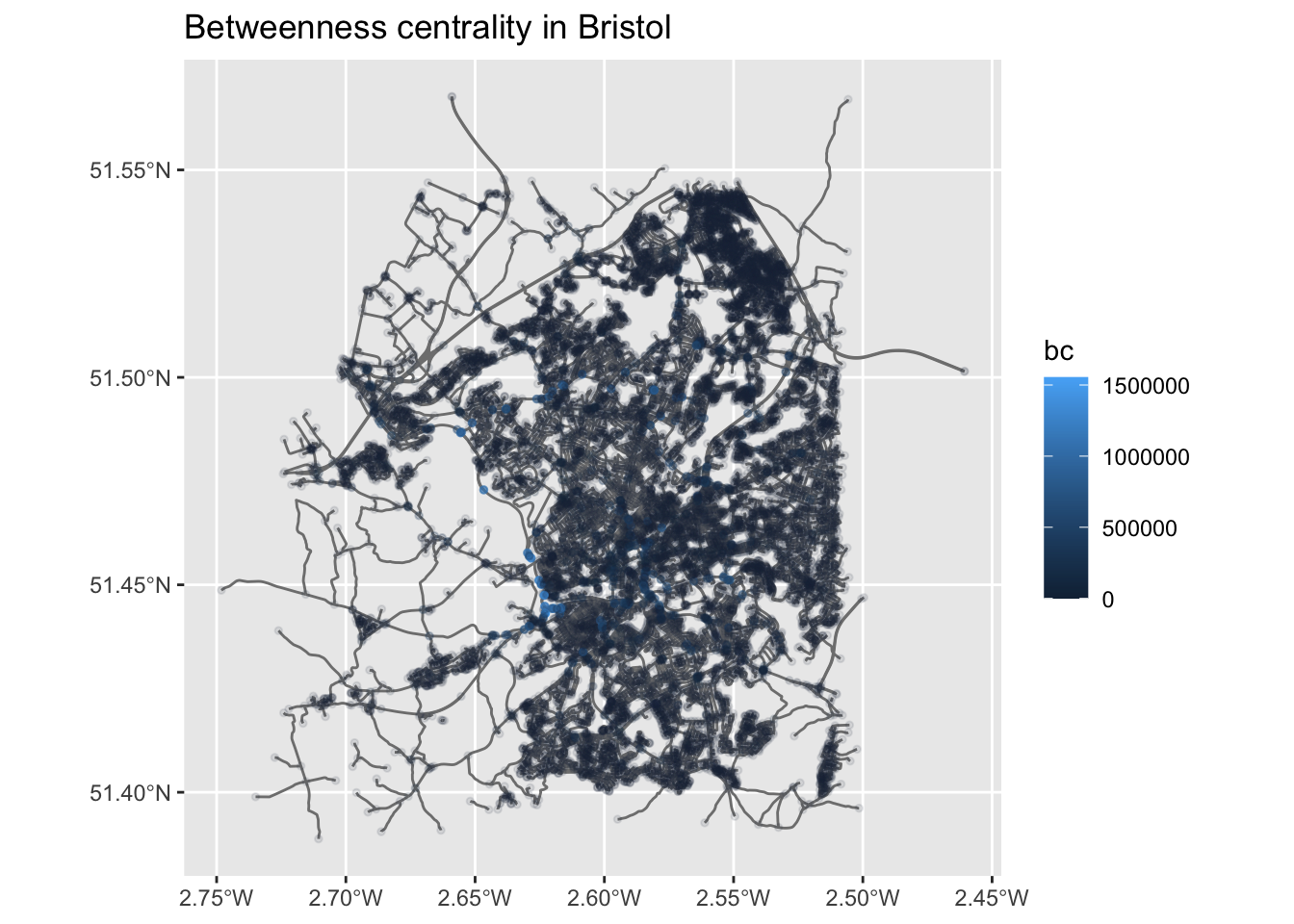Question: How can we interpret this map?

Remember the community detections algorithms? We can detect such communities within the street network. These are neighbourhoods of streets which are better connected internally than externally with the whole network. There is a contradiction here, no? Community detection is about nodes, not edges? However, here we are interested in clustering edges. So, we wiil do a trick. We first ‘inverse’ our spatial network, which means that the nodes become edges and vice versa. We do this with `morph(to_linegraph)`. Read the Wikipedia entry of line graps and see teh below image for an example. The `unmorgh()` brings the changes – that is the new `group` column back to the original network, that we now call `grouped_net`. For more details, have a look here.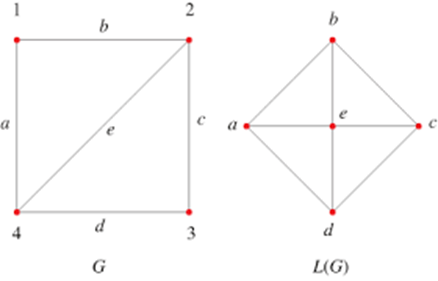Source: mathworld.wolfram.com

``````grouped_net = bristol_wrangled %>%
morph(to_linegraph) %>%
mutate(group = group_louvain()) %>%
unmorph()

# A new group column has been added with the community membership
grouped_net``````
``````# A sfnetwork with 17758 nodes and 11747 edges
#
# CRS:  EPSG:4326
#
# An undirected multigraph with 6657 components with spatially explicit edges
#
# Node Data:     17,758 x 2 (active)
# Geometry type: POINT
# Dimension:     XY
# Bounding box:  xmin: -2.748173 ymin: 51.38876 xmax: -2.460661 ymax: 51.56766
x     bc
<POINT [°]>  <dbl>
1  (-2.460661 51.5015)     0
2 (-2.521586 51.51787) 10561.
3 (-2.546315 51.54446)     0
4 (-2.552245 51.40075)  6653
5 (-2.516866 51.51245)  5452.
6 (-2.518981 51.51272) 31706.
# ... with 17,752 more rows
#
# Edge Data:     11,747 x 5
# Geometry type: LINESTRING
# Dimension:     XY
# Bounding box:  xmin: -2.748173 ymin: 51.38876 xmax: -2.460661 ymax: 51.56766
from    to weight                                                     x group
<int> <int>  <dbl>                                      <LINESTRING [°]> <int>
1     6     7 219.   (-2.518981 51.51272, -2.518938 51.51293, -2.518937 5~    28
2     8     9 980.   (-2.523849 51.52109, -2.522684 51.5198, -2.521651 51~    28
3    18    19   5.23              (-2.528247 51.50576, -2.528179 51.50578)    28
# ... with 11,744 more rows``````
``````# There are quite a few communities overall
grouped_net %>%
activate("edges") %>%
pull(group) %>%
unique() %>%
length()``````
`` 6699``
``````grouped_net %>%
activate("edges") %>%
st_as_sf() %>%                                     # If you remember, we used this above.
transmute(group = as.factor(group)) %>%            # We turn community membership to a factor.
filter(group %in% c(1:15)) %>%                     # Only plotting the first 15 communities.
ggplot() +
geom_sf(aes(col = group), lwd = 4) +               # This plots the communities ...
geom_sf(data = st_as_sf(bristol_wrangled, "edges"),# ... and this all the network.
col = "grey", lwd = 0.5, alpha = 0.4) +
theme(legend.position="none") +
ggtitle("Bristol's street communities")            # No legend please.``````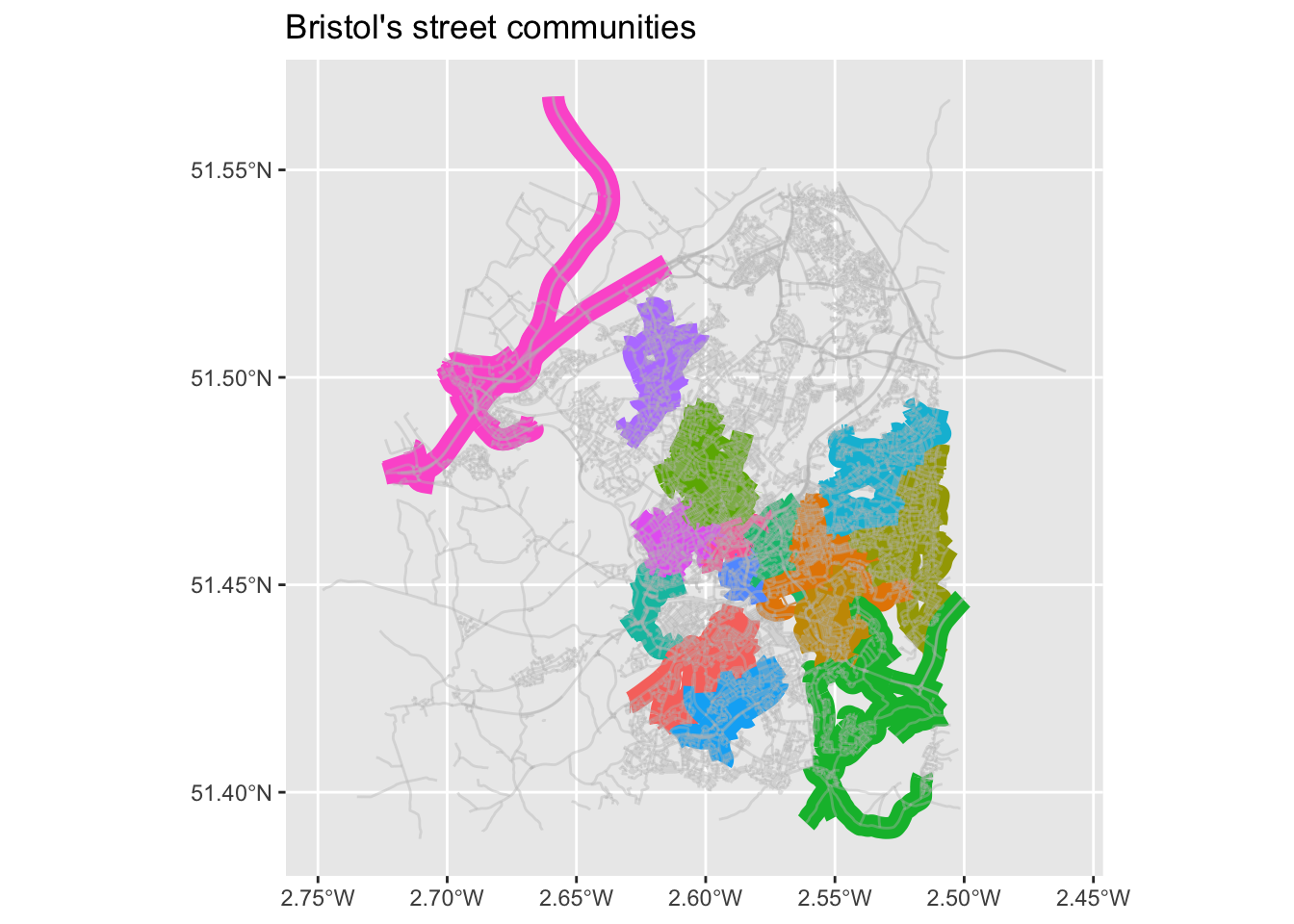Task: Read the routing vigneette of `sfnetworks and apply these techniques in the Bristol street network.

## References

Boeing, Geoff. 2017. “OSMnx: New Methods for Acquiring, Constructing, Analyzing, and Visualizing Complex Street Networks.” Computers, Environment and Urban Systems 65: 126–39.
———. 2019. “Urban Spatial Order: Street Network Orientation, Configuration, and Entropy.” Applied Network Science 4 (1): 1–19.
Haklay, Mordechai, and Patrick Weber. 2008. “Openstreetmap: User-Generated Street Maps.” IEEE Pervasive Computing 7 (4): 12–18.
Haklay, Muki, Dilek Fraisl, Bastian Greshake Tzovaras, Susanne Hecker, Margaret Gold, Gerid Hager, Luigi Ceccaroni, et al. 2021. “Contours of Citizen Science: A Vignette Study.” Royal Society Open Science 8 (8): 202108.
Hillier, Bill, Adrian Leaman, Paul Stansall, and Michael Bedford. 1976. “Space Syntax.” Environment and Planning B: Planning and Design 3 (2): 147–85.